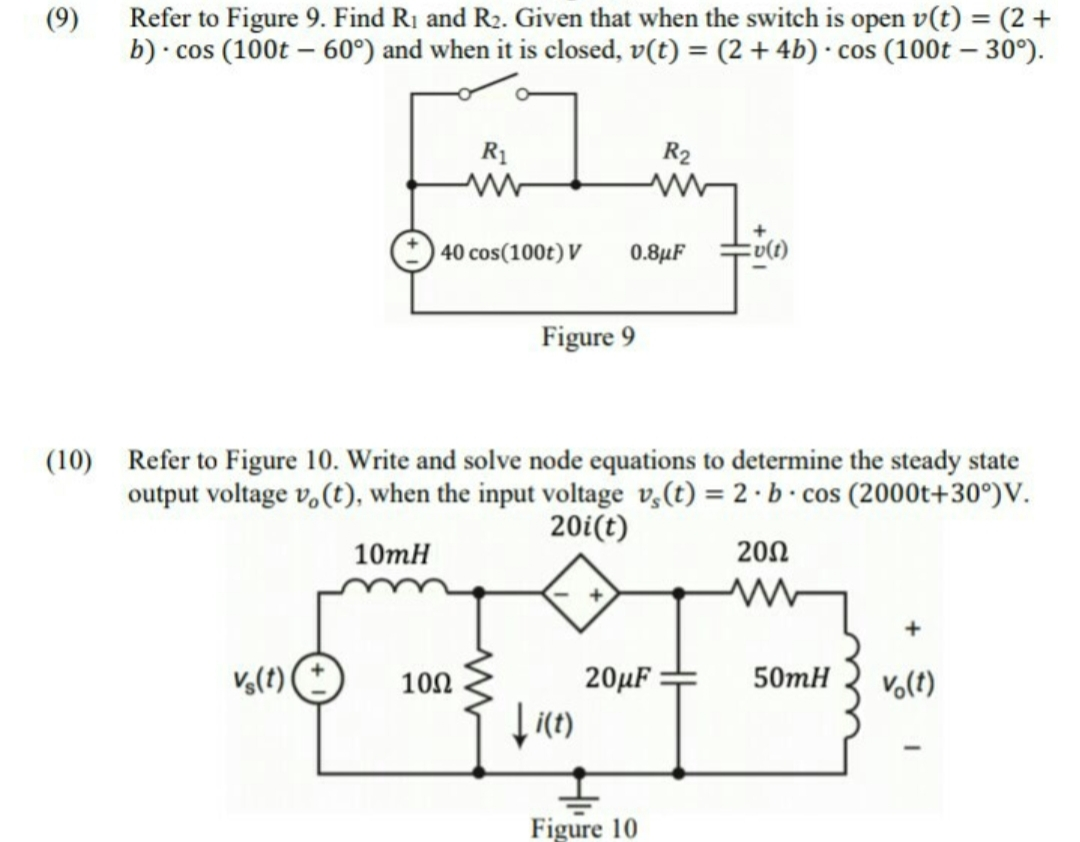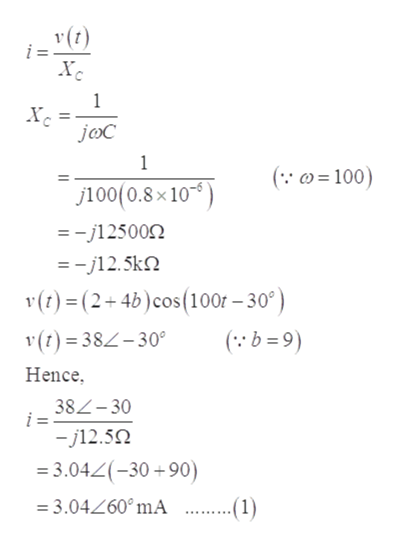# Refer to Figure 9. Find Ri and R2. Given that when the switch is open v(t)(9)(2cos (100t 30°)b) cos (100t 60°) and when it is closed, v(t) = (2 + 4b)R2R1:v(t)40 cos(100t) V0.8μFFigure 9(10)Refer to Figure 10. Write and solve node equations to determine the steady stateoutput voltage vo(t), when the input voltage v,(t) 2.b cos (2000t+300)V.20i(t)20Ω10mHwW50mHvs(t)20μFVolt)100Figure 10

Question
30 views

if the value needed,
a : 9
b : 9help_outlineImage TranscriptioncloseRefer to Figure 9. Find Ri and R2. Given that when the switch is open v(t) (9) (2 cos (100t 30°) b) cos (100t 60°) and when it is closed, v(t) = (2 + 4b) R2 R1 :v(t) 40 cos(100t) V 0.8μF Figure 9 (10) Refer to Figure 10. Write and solve node equations to determine the steady state output voltage vo(t), when the input voltage v,(t) 2.b cos (2000t+300)V. 20i(t) 20Ω 10mH wW 50mH vs(t) 20μF Volt) 100 Figure 10 fullscreen
check_circle

Step 1

Since we are entitled to answer one question, we’ll answer the first one. Please resubmit the question and specify the other question you’d like to get answered.

Step 2

Evaluating the value of resistances.

Firstly, close the switch to evaluate the value of resistance R2.

As the switch is closed, the resistance parallel to it will behave as a short-circuit and no current will flow through them.

Circuit diagram is shown below:

Step 3

Referring the question, the value of b is given as 9.

Now evalua...help_outlineImage Transcriptioncloseхе Хе joC 1 ( 100) j100(0.8x 10 =-j125000 j12.5k (t)= (2+4b) cos(100r- 30° ) v(t)38-30 Hence (b=9) 38L-30 i = j12.50 3.042(-30+90) = 3.04260°mA ...1) fullscreen

### Want to see the full answer?

See Solution

#### Want to see this answer and more?

Solutions are written by subject experts who are available 24/7. Questions are typically answered within 1 hour.*

See Solution
*Response times may vary by subject and question.
Tagged in

### Electrical Engineering